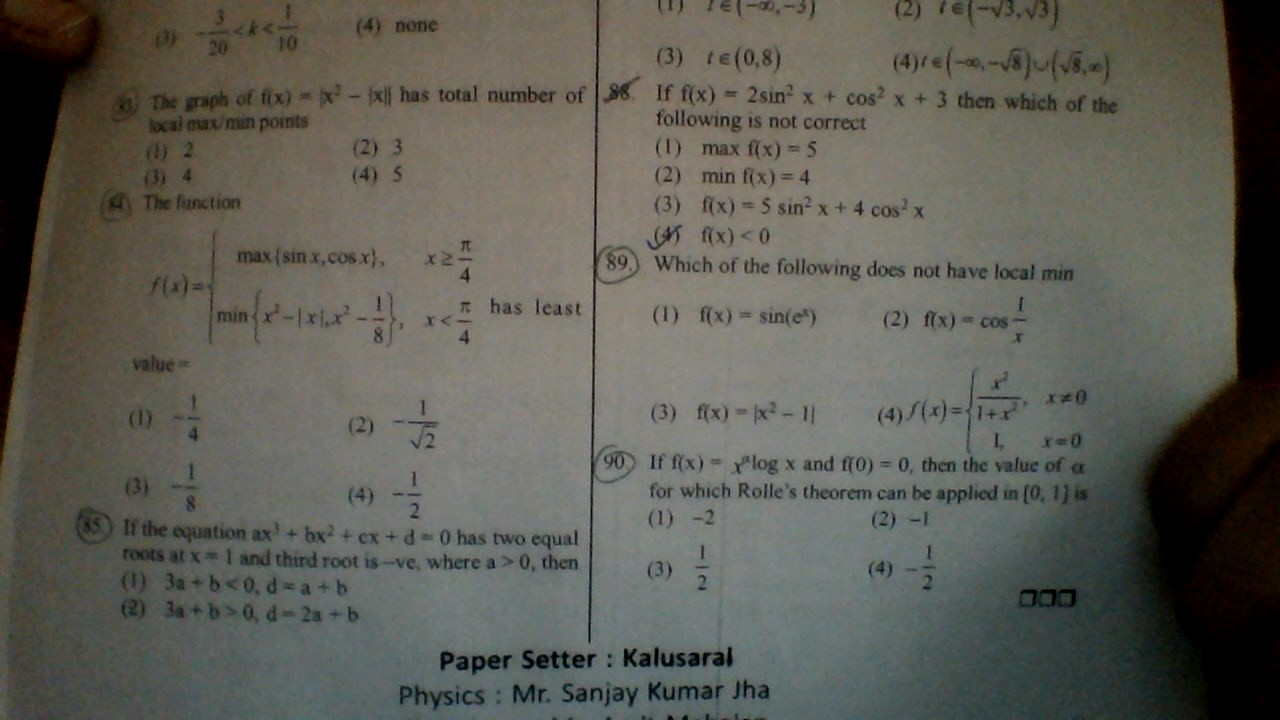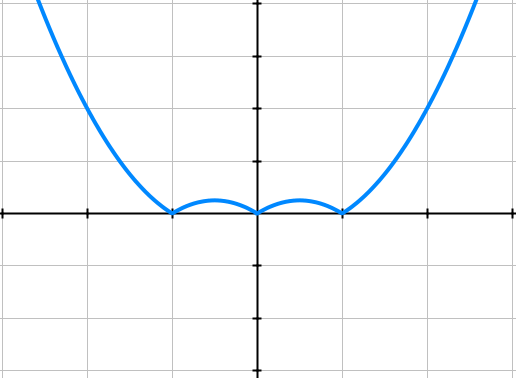•Manish Shankar ·

The graph will look like thisWhich shows it has 5 critical point

Amartya Kumar Mistry ·

drawing the graph i am getting option c for the q. 83

Amartya Kumar Mistry ·

for q. 89 ans is option 2

Himanshu Giria ·

84 .(2)

Himanshu Giria ·

In 90 i guess Î± can have any real value so I guess all the4options are correct
...

man111 singh ·

\hspace{-16}$For$\bf{(85)::}$Given$\bf{ax^3+bx^2+cx+d = a(x-1)^2\cdot (x-\gamma).........(1)}$\\\\\\ Where$\bf{a>0}$and$\bf{\gamma<0}$, Now put$\bf{x=1}$on both side, we get\\\\\\$\bf{\Rightarrow a+b+c+d = 0\Rightarrow c=-\left(a+b+d\right)}$\\\\\\ Now Diif. equation$\bf{(1)}$both side w. r to$\bf{x\;,}$we get\\\\\\$\bf{\Rightarrow 3ax^2+2bx+c = a\left\{(x-1)^2+2(x-1)(x-\gamma)\right\}}$\\\\\\ Now put$\bf{x=1}$on both side, we get\\\\\\$\bf{\Rightarrow 3a+2b+c = 0\Rightarrow 3a+2b-a-b-d = 0\Rightarrow \boxed{\boxed{\bf{2a+b=d}}}}$\\\\\\ (Using$\bf{c=-\left(a+b+d\right)}$)\\\\\\ Now Using Product of Roots for equation$\bf{(1)\;,}$We get\\\\\\$\bf{\Rightarrow \gamma = -\frac{d}{a}\Rightarrow d=-\gamma \cdot a >0}$\\\\\\ So$\bf{d=2a+b>0}$\\\\\\ So$\bf{\boxed{\boxed{\bf{d+a= 2a+b+b>0\Rightarrow 3a+b>0}}}}$\\\\\\ bcz$\bf{a\;,d >0}\$

•